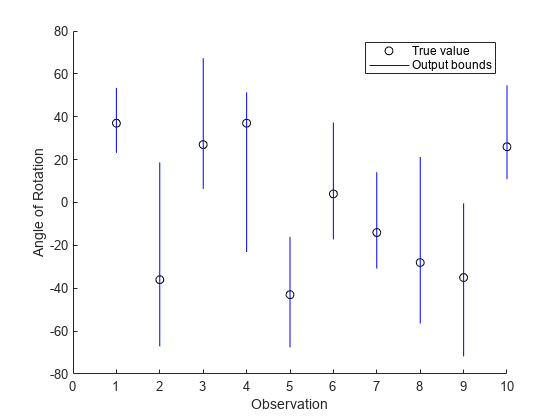# estimateNetworkOutputBounds

Estimate output bounds of deep learning network

Since R2022b

## Syntax

``[YLower,YUpper] = estimateNetworkOutputBounds(net,XLower,XUpper)``

## Description

example

````[YLower,YUpper] = estimateNetworkOutputBounds(net,XLower,XUpper)` estimates the lower and upper output bounds, `YLower` and `YUpper`, respectively, of the network `net` for input within the bounds specified by `XLower` and `XUpper`. The function estimates the range of output values that the network returns when the input is between the specified lower and upper bounds. Use this function to estimate how sensitive the network predictions are to input perturbation. The `estimateNetworkOutputBounds` function requires the Deep Learning Toolbox Verification Library support package. If this support package is not installed, use the Add-On Explorer. To open the Add-On Explorer, go to the MATLAB® Toolstrip and click Add-Ons > Get Add-Ons.```

## Examples

collapse all

Estimate the output bounds for an image regression network.

Load a pretrained regression network. This network is a `dlnetwork` object that has been trained to predict the rotation angle of images of handwritten digits.

`load("digitsRegressionNetwork.mat");`

`[XTest,~,TTest] = digitTest4DArrayData;`

Select the first ten images.

```X = XTest(:,:,:,1:10); T = TTest(1:10);```

Convert the test images to `dlarray` objects.

`X = dlarray(X,"SSCB");`

Estimate the output bounds for an input perturbation between –0.01 and 0.01 for each pixel. Create lower and upper bounds for the input.

```perturbation = 0.01; XLower = X - perturbation; XUpper = X + perturbation;```

Estimate the output bounds for each input.

`[YLower,YUpper] = estimateNetworkOutputBounds(net,XLower,XUpper);`

The output bounds are `dlarray` objects. To plot the output bounds, first extract the data using `extractdata`.

```YLower = extractdata(YLower); YUpper = extractdata(YUpper);```

Visualize the output bounds.

```figure hold on for i = 1:10 plot(i,T(i),"ko") line([i i],[YLower(i) YUpper(i)],Color="b") end hold off xlim([0 10]) xlabel("Observation") ylabel("Angle of Rotation") legend(["True value","Output bounds"])```## Input Arguments

collapse all

Network, specified as an initialized `dlnetwork` object. To initialize a `dlnetwork` object, use the `initialize` function.

The function supports networks with these layers:

The function does not support networks with multiple inputs and multiple outputs.

The function estimates the output bounds using the final layer in the network. For most applications, use the final fully connected layer when computing the output bounds. If your network has a different layer as its final layer, remove the layer before calling the function.

Input lower bound, specified as a formatted `dlarray` object. For more information about `dlarray` formats, see the `fmt` input argument of `dlarray`.

The lower and upper bounds, `XLower` and `XUpper`, must have the same size and format. The function computes the results across the batch (`"B"`) dimension of the input lower and upper bounds.

Input upper bound, specified as a formatted `dlarray` object. For more information about `dlarray` formats, see the `fmt` input argument of `dlarray`.

The lower and upper bounds, `XLower` and `XUpper`, must have the same size and format. The function computes the results across the batch (`"B"`) dimension of the input lower and upper bounds.

## Output Arguments

collapse all

Output lower bound, returned as a formatted `dlarray` object. For more information about `dlarray` formats, see the `fmt` input argument of `dlarray`.

The function estimates the output bounds for each observation across the batch (`"B"`) dimension. If you supply `k` upper bounds and lower bounds, then `YLower` contains `k` output lower bounds. For more information, see Algorithms.

Output upper bound, returned as a formatted `dlarray` object. For more information about `dlarray` formats, see the `fmt` input argument of `dlarray`.

The function estimates the output bounds for each observation across the batch (`"B"`) dimension. If you supply `k` upper bounds and lower bounds, then `YUpper` contains `k` output upper bounds. For more information, see Algorithms.

## Algorithms

Let X be the input for which you want to estimate the output bounds. To use the `estimateNetworkOutputBounds` function, you must specify a lower and upper bound for the input. For example, let $ϵ$ be a small perturbation. You can define a lower and upper bound for the input as ${X}_{\text{lower}}=X-ϵ$ and ${X}_{\text{upper}}=X+ϵ$, respectively.

To estimate the output bounds for the network `net` and the input bounds Xlower and Xupper, the function performs these steps.

1. Create an input set using the lower and upper input bounds.

2. Pass the input set through the network and return an output set. To reduce computational overhead, the function performs abstract interpretation by approximating the output of each layer using the DeepPoly  method.

3. Return the minimum and maximum estimated output values for the input set.

If you specify multiple pairs of input lower and upper bounds, then the function estimates the output bounds for each pair of input bounds.

Note

Because of floating-point round-off error, the output bounds might be slightly different when working with networks produced using C/C++ code generation.

 Goodfellow, Ian J., Jonathon Shlens, and Christian Szegedy. “Explaining and Harnessing Adversarial Examples.” Preprint, submitted March 20, 2015. https://arxiv.org/abs/1412.6572.

 Singh, Gagandeep, Timon Gehr, Markus Püschel, and Martin Vechev. “An Abstract Domain for Certifying Neural Networks”. Proceedings of the ACM on Programming Languages 3, no. POPL (January 2, 2019): 1–30. https://doi.org/10.1145/3290354.